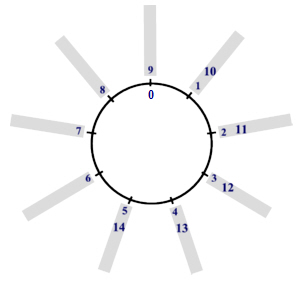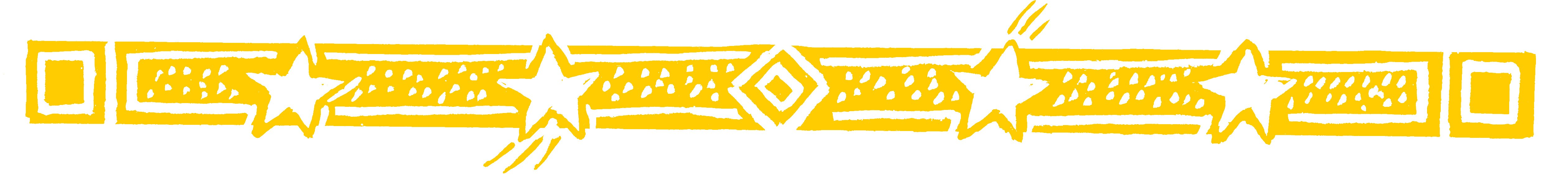THE NINE-POINT CIRCLE

Since all numbers, no matter how long, can be reduced to a single figure every number finds its place on the nine-point circle:This circle shows the numbers from 1 to 9 and continuing to number round the circle would put 10 at the same place as 1. 11 would be at the same place as 2 and so on as shown below:Notice also that 0 goes at the same place as 9 because, numbering backwards round the circle, 0 comes before 1.

So all numbers will have their place somewhere round this circle.

Now, looking at the last circle, the digit sum of 10 is 1, and 10 is next to 1 on the circle.
The digit sum of 11 is 2, and 11 is next to 2.
And so on.

To find which branch of the circle a number is on we simply find its digit sum.

• For example the digit sum of 34 is 7 so 34 will come on the ‘7 branch’.

You could check this by continuing to number
round the circle until you get to 34.

• 77 will be on the ‘5 branch’ as 77=14=5.

Question Comment !
 Tutorial Title Question Title No Questions 0 Questions Asked 0 Skipped Questions 0 Correctly Answered 0 Wrongly Answered 0 Total Question Attempts 0 Current Question Attempts 0

Skip Question

Reset Test

Halt Test

 No Questions 0 Questions Asked 0 Skipped Questions 0 Correctly Answered 0 Wrongly Answered 0 Total Question Attempts 0

Time Taken

CloseThe Nine Point Circle